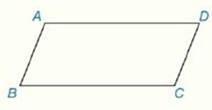Chapter 4.CR, Problem 13CR### Elementary Geometry for College St...

6th Edition
Daniel C. Alexander + 1 other
ISBN: 9781285195698

#### Solutions

Chapter
Section### Elementary Geometry for College St...

6th Edition
Daniel C. Alexander + 1 other
ISBN: 9781285195698
Textbook Problem
9 views

# Review Exercises Given: ▱ A B C D C D = 2 x + 3 B C = 5 x - 4 Perimeter of ▱ A B C D = 96 cm Find: The lengths of the sides of ▱ A B C DTo determine

To Find:

The side lengths of the provided parallelogram ABCD.

Explanation

Formula Used:

The perimeter of a polygon is the sum of the lengths of all sides of the polygon.

That is, Perimeter of the parallelogram P=2(l+b), where l is the length and b is the base of the parallelogram.

Calculation:

It is given that ABCD is a parallelogram with CD=2x+3 and BC=5x-4.

Also provided that the perimeter of the parallelogram ABCD,  P=96 cm.

In the parallelogram ABCD, AD is parallel to BC and AB is parallel to CD. That is, ADBC and ABCD. Also they are equal in measure. That is, AD=BC and AB=CD

### Still sussing out bartleby?

Check out a sample textbook solution.

See a sample solution

#### The Solution to Your Study Problems

Bartleby provides explanations to thousands of textbook problems written by our experts, many with advanced degrees!

Get Started

#### In Problems 19-44, factor completely. 36.

Mathematical Applications for the Management, Life, and Social Sciences

#### Simplify: 1339

Elementary Technical Mathematics

#### Convert the expressions in Exercises 6584 to power form. x2y

Finite Mathematics and Applied Calculus (MindTap Course List)